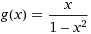# Find the range of this function

## Homework Statement

A function g is defined by, x=/= +-1 . Determine the range of of g.

## The Attempt at a Solution

Is it {y:y∈R} or {y:y∈R, y=/=0}? Is ''0'' included? Because x=0 is the asymptote of the function but at the same time, function g also intersect the y-axis at (0,0). So i am confused on whether the answer should include 0 or not... Can anyone enlighten me? Thanks.

## Answers and Replies

mege
x=0 is not an a asymptote.

You already know that x=1 and x=-1 cause the function to divide by zero - what happens immediately on either side of x=1 and -1?

eumyang
Homework Helper
I'm guessing that the OP should have meant "y = 0" as an asymptote, not "x = 0."

A function can intersect its horizontal asymptote in the "middle" of the graph. Just not at the "ends" -- when x approaches infinity and/or negative infinity.

hunt_mat
Homework Helper
Right, you know that when 0<x<1 this means that 1-x^2>0 and therefore, g will be positive, for 0>x>-1, g(x) will be negative. Using this, examine when x>1 and x<-1.